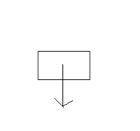# Vocabulary Newton’s Laws

Force-any push or pull on an object

Free body diagrams-diagrams used to show the magnitude and direction of all forces acting on an object.

Normal force-the force that acts on a surface in a direction perpendicular to the surface

Weight-the gravitational force exerted on an object.

Newton’s First Law of Motion-An object at rest or in motion will remain in that state unless an unbalanced force acts on the object.

Newton’s Second Law of Motion-The net force on an object is equal to the mass times the acceleration.

Newton’s Third Law of Motion-For every action, there is an equal and opposite reaction.

Net force-The sum of all the forces acting on an object.

Equilibrium-the condition in which the net force on an object is zero.

Newton’s Law of Gravitation-states that every particle in the universe attracts every other with a force that is proportional to the product of their masses and inversely proportional to the square of distance between them.

For the next ones, write your own definition based on your understanding after reading the description and looking at the image.

Weight (W or FW or Fg = mg). All bodies have mass and therefore have a weight. Find this by multiplying the mass (in kg) by the acceleration due to gravity (g = 9.8 m/s2). On the AP exam, it is perfectly acceptable to use a value of 10 m/s2 for the sake of time. Even though g is a negative value, weights are not expressed as negative, but the vector always points down toward the bottom of your page.Normal (N or FN). The normal is directed upwards perpendicular to the plane of motion. Sometimes, but not always, it is equal to the weight…only if angles are NOT involved. Consider a box sitting on a frictionless floor:Applied Force (F or FA). This is the force (push or pull) being applied to the object. This is NOT the net force! Consider a box that is being pushed along a frictionless surface:Friction (f or Ff). This force opposes motion.   It can be to the right, left, up the ramp, down the ramp, whichever is the opposite direction to the motion. Consider the same box as above, but now it is being pushed on a surface which has friction.

Notice in this diagram that the friction vector is smaller than the applied vector. This would indicate that the net force (and therefore acceleration) would be to the right. Notice that the normal and weight, though, are (roughly) the same length. In this example, the normal would equal the weight because there was no acceleration vertically .Tension (T or FT). This is the force transmitted by a string. Any dynamics problem that has a string involved, massless or otherwise, will have a tension force associated with it. For example, see the diagram.

Notice that the weight is always present and that there are two tension forces with associated angles. This diagram would be appropriate for a statics problem. You will see these later in this unit.(source)